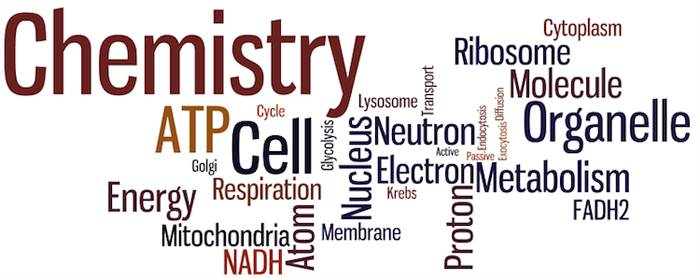Other Entrance Exams

Optical Isomerism in Coordination CompoundsOptical Isomerism Questions:

Ques. In coordination compound [Co(en)2Cl2]Cl which is false
(a) Show geometrical Isomerism
(b) Show optical Isomerism
(c) Show ionic Isomerism
(d) A octahedral complex
Ans. (c)

Ques. [Co(NH3)4Cl2]+ exhibits
(a) Geometrical isomerism
(b) Optical isomerism
(c) Bonding isomerism
(d) Ionisation isomerism
Ans. (a)

Ques. The number of unpaired electrons in Ni(CO)4 is
(a) Zero
(b) One
(c) Three
(d) Five
Ans. (a)

Ques. The possible number of optical isomers in [Co(en)2Cl2]+ are
(a) 2
(b) 3
(c) 4
(d) 6
Ans. (b)

Ques. The colour of CoCl3.5NH3.H2O is
(a) Orange yellow
(b) Orange
(c) Green
(d) Pink
Ans. (d)

Related: Optics question bank

Ques. Paramagnetic co-ordination compounds contain …….. electrons
(a) No
(b) Both paired and unpaired
(c) Paired
(d) Unpaired
Ans. (d)

Ques. The type of magnetism exhibited by [Mn(H2O)6]2+ ion is
(a) Paramagnetism
(b) Diamagnetism
(c) Both (a) and (b)
(d) None of these
Ans. (a)

Ques. Coordination isomerism is caused by the interchange of ligands between the
(a) Cis and Trans structure
(b) Complex cation and complex anion
(c) Inner sphere and outer sphere
(d) Low oxidation and higher oxidation states
Ans. (a)

Ques. What is the magnetic moment of K3[FeF6]
(a) 5.91 BM
(b) 4.89 BM
(c) 3.87 BM
(d) 6.92 BM
Ans. (a)

Ques. [Co(NH3)5Br]SO4 and [Co(NH3)5SO4] are examples of which type of isomerism
(b) Geometrical
(c) Ionization
(d) Optical
Ans. (c)

Ques. Types of isomerism shown by [Cr(NH3)5NO2]Cl2 is
(a) Optical
(b) Ionisation
(c) Geometrical
Ans. (d)

Related: thermodynamics quiz

Ques. [Co(NH3)5NO2]Cl2 and [Co(NH3)5(ONO)]Cl2 are related to each other as
(a) Geometrical isomers
(b) Optical isomers
(d) Coordination isomers
Ans. (c)

Ques. If the magnetic moment of [Ag(CN)2]–1 is zero, then the number of unpaired electrons will be
(a) 1
(b) 2
(c) 3
(d) Zero
Ans. (d)

Ques. Which one of the following octahedral complexes will not show geometric isomerism (A and B are monodentate ligands)
(a) [MA5B]
(b) [MA2B4]
(c) [MA3B3]
(d) [MA4B2]
Ans. (a)

Ques. The complexes [Co(NH3)6][Cr(C2O4)3] and [Cr(NH3)6][Co(C2O4)3]
(b) Geometrical isomerism
(c) Coordination isomerism
(d) Ionisation isomerism
Ans. (c)

Ques. The number of geometrical isomers for [Pt(NH3)2Cl2] is
(a) Two
(b) One
(c) Three
(d) Four
Ans. (a)

Ques. The number of ions per mole of a complex [CoCl2.5NH3]Cl2 in aqueous solution will be
(a) Nine
(b) Four
(c) Three
(d) Two
Ans. (c)

Ques. [Ti(H2O)6]+3  is paramagnetic in nature due to
(a) One unpaired e
(b) Two unpaired e
(c) Three unpaired e
(d) No unpaired e
Ans. (a)

Ques. Which of the following coordiantion compounds would exhibit optical isomerism
(a) trans-dicyanobis (ethylenediamine) chromium (III) chloride
(b) tris-(ethylenediamine) cobalt (III) bromide
(c) pentaamminenitrocobalt (III) iodide
(d) diamminedichloroplatinum (II)
Ans. (b)

Related: Current Electricity questions

Ques. How many ions will be produced in solution from one molecule of chloropentammine cobalt (III) chloride
(a) 1
(b) 2
(c) 3
(d) 4
Ans. (c)

Ques. The pair of complex compounds [Cr(H2O)6Cl3] and [Cr(H2O)5Cl]Cl2H2O are an example of
(b) Ionisation isomerism
(c) Coordination isomerism
(d) Hydrate isomerism
Ans. (d)

Ques. [Pt(NH3)4Cl2]Br2 and [Pt(NH3)4Br2]Cl2 are related to each other as
(a) Optical isomers
(b) Coordinate isomers
(c) Ionization isomers
Ans. (c)

Related: environmental pollution questions and answers

Ques. What is true for [Fe(CN)6]3– and [FeF6]3–
(a) Both are paramagnetic
(b) Only [Fe(CN)6]3– is paramagnetic
(c) Only [FeF6]3–is paramagnetic
(d) Both are diamagnetic
Ans. (a)